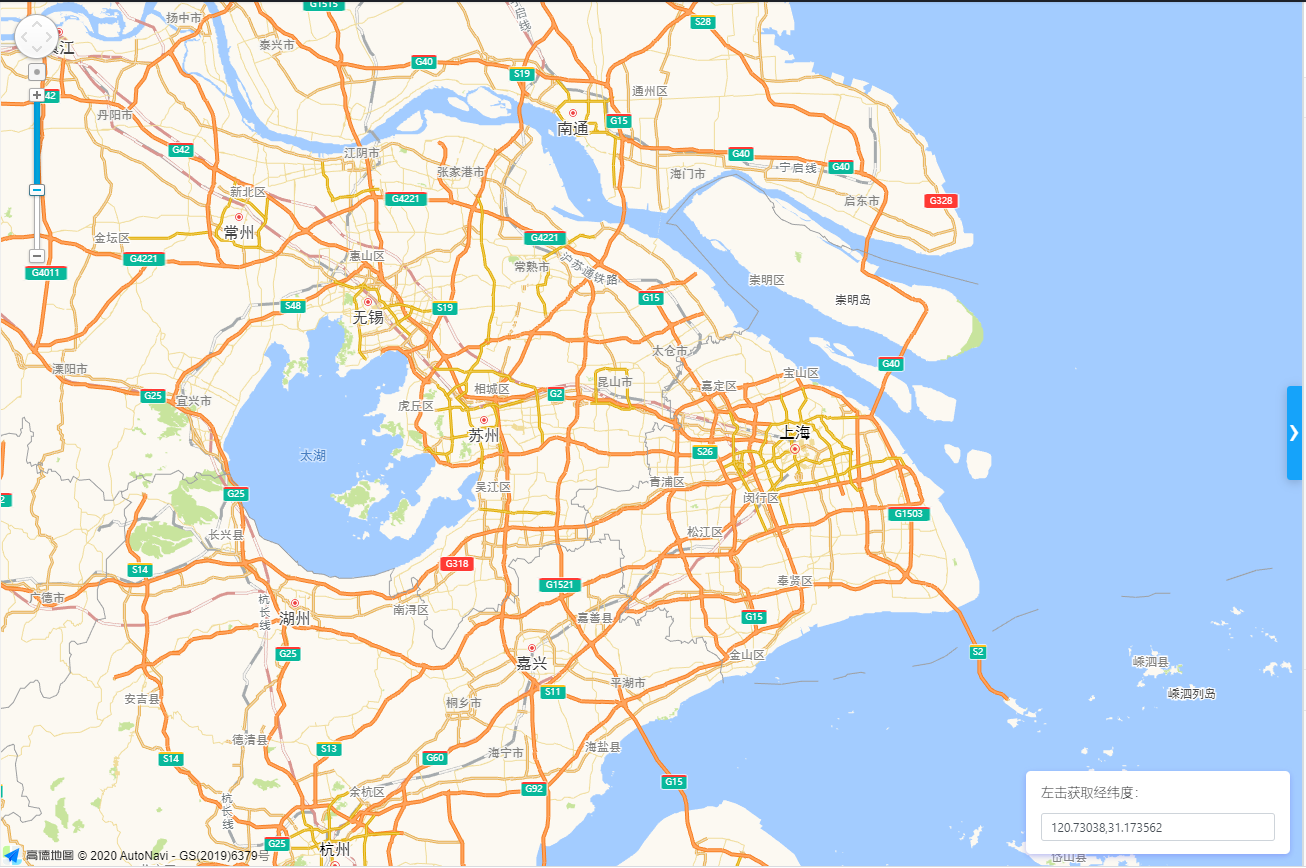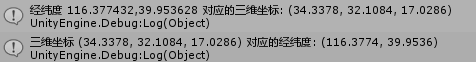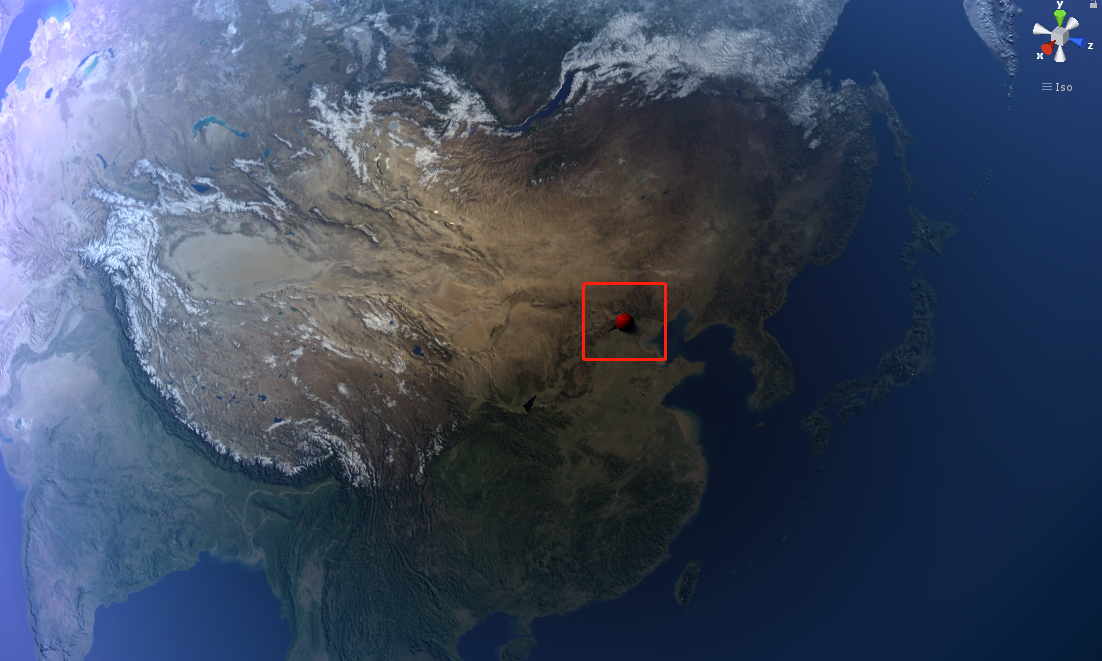# Unity3D离线版数字地球实现

## 概述

### 效果展示### 设计思路

• 首先找个高清点的地球三维模型（这里是我上某宝买的一个unity3d地球高清模型包）
• 然后找到不同级别的瓦片地图，这里稍微科普一下什么是瓦片地图：
• 参考资料：国内主要地图瓦片坐标系定义及计算原理
• 简单而言就是不同级别的瓦片数量不同，越高级别的瓦片数量越多，展示的地图越详细，某一瓦片等级地图的瓦片是由低一级的各瓦片切割成的4个瓦片组成，形成了瓦片金字塔。
• 所以离线版数字地球的话，最重要的也就是这个不同级别瓦片的获取方法了，可以联网的话，我们是可以去申请BingMap的Key在线获取这些瓦片地图资源的。这种Key如何使用，大家可以参考一下Unity一个叫做WorldComposer的插件(某宝也有卖，当然如果可以的话大家还是支持下正版比较好啊)，这个插件的使用教程Unity3D地形建模插件World Composer用法（大范围地形建模）
• 但是目前需求是要整一个离线版的数字地球，所以没办法我们只能预先把这些瓦片地图玩意给先下下来，然后在本地去进行加载了。至于怎么搞到这些离线的瓦片地图资源: 全球瓦片数量贼多，一般想要白嫖到这些资源感觉还是有点难度的，也试了一下什么水经注，GlobalMap等一些GIS软件，好像不是要收费就是各种限制，软件入门也有点难搞。所以这里我又去某宝搜了一下，花了我几百大洋(心痛！)，找了一个店家买了全球0-9级的瓦片，中国区域0-12级的瓦片资源。
• OK，地球模型有了，离线瓦片资源也有了的情况下，我们就可以进行离线版数字地球的开发了。

### GIS相关计算公式(经纬度与三维坐标)

• 根据经纬度转三维坐标：我们需要根据给定的一个经纬度坐标，得到其在Unity世界三维点的位置：
	/// <summary>
/// 根据经纬度计算球面坐标
/// </summary>
/// <param name="longitude">经度</param>
/// <param name="latitude">纬度</param>
/// <returns></returns>
private Vector3 GetSphericalCoordinates(double longitude,double latitude)
{
latitude = latitude * Mathf.PI / 180D;
longitude = longitude * Mathf.PI / 180D;
double x = EarthRadius * Mathf.Cos((float)latitude) * Mathf.Sin((float)longitude);
double y = EarthRadius * Mathf.Sin((float)latitude);
double z = -EarthRadius * Mathf.Cos((float)latitude) * Mathf.Cos((float)longitude);
return new Vector3((float)x, (float)y, (float)z);
}

• 以及对应的逆变换:根据三维坐标活得其经纬度,这里要注意一下经度范围是-180到180，纬度是-90到90，注意下程序里面正负号就行了。
	/// <summary>
/// 根据三维坐标计算经纬度
/// </summary>
/// <param name="pos">目标三维坐标</param>
/// <returns></returns>
private Vector2 CalculateLontitudeAndLatitude(Vector3 pos)
{
int s = 1;
int q = 1;
if (pos.x < 0.0f)
{
s = -1;
}
if(pos.y > 0.0f)
{
q = -1;
}
float latitudeAngle = Mathf.Asin(pos.y / EarthRadius);
float latitude = latitudeAngle * 180.0f / Mathf.PI;

float longtitudeAngle = Mathf.Acos(pos.z / (-1.0f * EarthRadius * Mathf.Cos(latitudeAngle)));
float longtitude = longtitudeAngle * 180.0f / Mathf.PI;
return new Vector2(s * longtitude, q * latitude);
}

• 简单验证一下:
可以从高德地图这里随便点一个点的位置，然后获取这个地方的经纬度把这经纬度输入unity里面进行计算一下，比如这里我以北京经纬度(116.541843,39.661317)为例:简单的目测位置好像差不太多,可能因为精度问题会有一些偏差，这个之后再去整理一下吧。

### GIS计算公式(瓦片，像素与经纬度)

• 双曲函数，因为Mathf里面好像没有双曲函数Sinh，所以这里简单写一个双曲函数的计算
	/// <summary>
/// 双曲函数
/// </summary>
/// <returns></returns>
private float Sinh(float x)
{
float ax = (Mathf.Exp(x) - Mathf.Exp(-1.0f * x))/2;
return ax;
}

• 根据经纬度获取目标瓦片位置坐标
	/// <summary>
/// 根据经纬度获取目标瓦片位置
/// </summary>
/// <param name="longtitude">经度</param>
/// <param name="latitude">纬度</param>
/// <returns></returns>
private Vector2 CalculateTileIndex(double longtitude,double latitude)
{
int x = (int)(Mathf.Pow(2, ZoomLevel - 1) * (longtitude / 180 + 1));
int y = (int)(Mathf.Pow(2, ZoomLevel - 1) * (1 - Mathf.Log((Mathf.Tan(Mathf.PI * (float)latitude / 180) + 1/Mathf.Cos(Mathf.PI * (float)latitude / 180)), 2.7182818f) / Mathf.PI));
return new Vector2(x, y);
}

• 根据瓦片编号获取经纬度信息
	/// <summary>
/// 根据瓦片编号获取经纬度信息
/// </summary>
/// <param name="xtile">瓦片x编号</param>
/// <param name="ytile">瓦片y编号</param>
/// <returns></returns>
private Vector2 CalculateLongAndLa(int xtile,int ytile)
{
float n = Mathf.Pow(2, ZoomLevel);
float longtitude = xtile / n * 360.0f - 180.0f;
float latitude = Mathf.Atan(Sinh(Mathf.PI * (1 - 2 * ytile / n))) * 180.0f / Mathf.PI;
return new Vector2(longtitude, latitude);
}

• 经纬度坐标转像素坐标
	/// <summary>
/// 经纬度坐标转像素坐标
/// </summary>
/// <param name="longtitude">经度</param>
/// <param name="latitude">纬度</param>
/// <returns>像素坐标值</returns>
private Vector2 LongAndLaToPixel(float longtitude,float latitude)
{
float pixelX = (longtitude + 180 / 360) * Mathf.Pow(2, ZoomLevel) * 256 % 256;
float pixelY = (1 - Mathf.Log(Mathf.Tan(latitude * Mathf.PI / 180) + 1 / (Mathf.Cos(latitude * Mathf.PI / 180)), 2.7182818f) / (2 * Mathf.PI)) * Mathf.Pow(2, ZoomLevel) * 256 % 256;
return new Vector2(pixelX, pixelY);
}

• 根据瓦片上某一点的像素坐标得到经纬度坐标
	/// <summary>
/// 根据瓦片上某一点的像素坐标得到经纬度坐标
/// </summary>
/// <param name="tileX">瓦片X编号</param>
/// <param name="tileY">瓦片Y编号</param>
/// <param name="pixelX">目标点X像素坐标</param>
/// <param name="pixelY">目标点Y像素坐标</param>
/// <returns></returns>
private Vector2 PixelToLongAndLa(float tileX,float tileY,float pixelX,float pixelY)
{
float longtitude = (tileX + pixelX / 256) / (Mathf.Pow(2, ZoomLevel)) * 360 - 180.0f;
float latitude = (Mathf.Asin(Sinh(Mathf.PI - 2 * Mathf.PI * (tileY + pixelY / 256) / (Mathf.Pow(2, ZoomLevel))))) * 180 / Mathf.PI;
return new Vector2(longtitude, latitude);
}


## 基本流程

• 根据摄像机与地球球心(这里我是用一个标准的球形代替了地球)的距离确定当前的瓦片等级
• 从摄像机位置朝地球球心发射一条射线，获取hit point三维坐标，转化位经纬度坐标再根据瓦片等级转化为得到该位置的目标瓦片位置
• 根据目标瓦片位置坐标获取其上下左右斜上斜下8个位置的瓦块信息并加载出来，加载结束。

## 瓦片资源包03-21
07-012135
09-06439
12-293万+
05-129220
08-08
01-08
01-16
07-151万+
11-03
11-03
01-301280
02-10

### “相关推荐”对你有帮助么？

•非常没帮助
•没帮助
•一般
•有帮助
•非常有帮助被折叠的  条评论 为什么被折叠?到【灌水乐园】发言nature_biubiu

¥1 ¥2 ¥4 ¥6 ¥10 ¥20获取中扫码支付点击重新获取扫码支付1.余额是钱包充值的虚拟货币，按照1:1的比例进行支付金额的抵扣。
2.余额无法直接购买下载，可以购买VIP、付费专栏及课程。余额充值